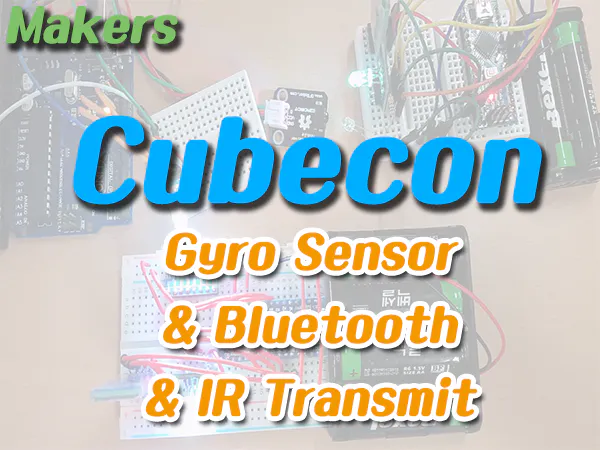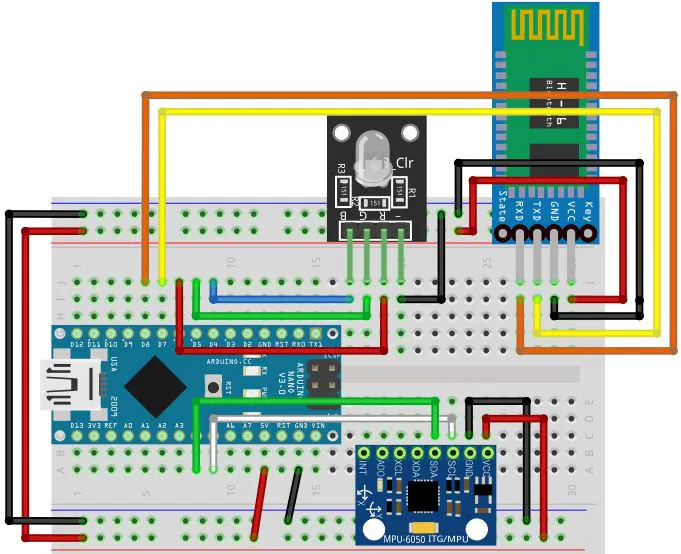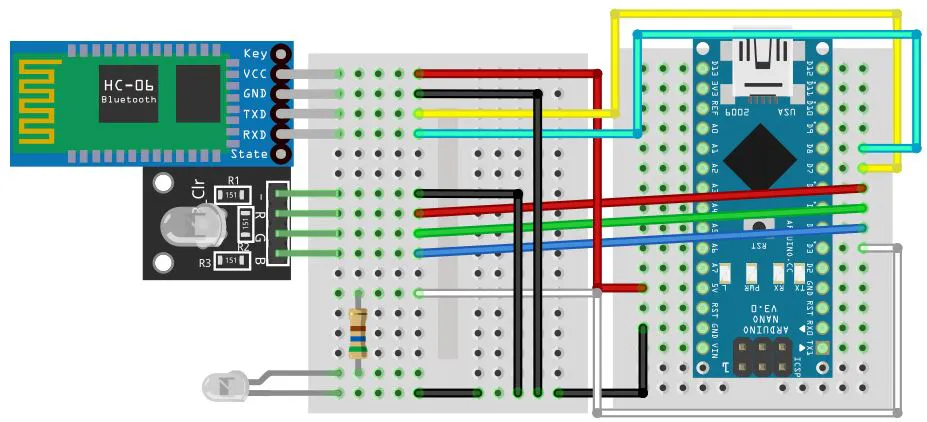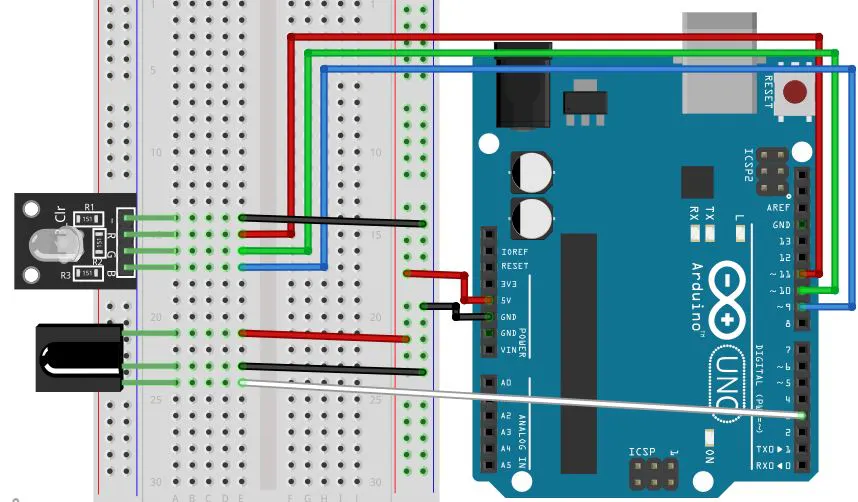Published

# Makers (Cubecon) #3 Gyro Sensor, Bluetooth & IR Transmit

A Bongilcheon High School Makers club's team project.

BeginnerWork in progress1 hour823## Things used in this project

### Hardware componentsArduino Nano R3
×3×3
 Arduino MPU-6050
×1
 HC-06 Bluetooth Module
×2
 Arduino RGB LED
×3
 Arduino Infrared transmitter
×1
×1

### Software apps and online servicesArduino IDE

## Schematics

### Gyro Sensor_Bluetooth_RGB LED### Infrared Transmit_Bluetooth## Code

### Gyro Sensor_Bluetooth_RGB LED

Arduino
```#include<Wire.h>
#include<SoftwareSerial.h>

SoftwareSerial btSerial(7, 8);

int16_t GyX, GyY, GyZ;
int red = 6;
int green = 5;
int blue = 4;

void setup() {
Wire.begin();
Wire.write(0x6B);
Wire.write(0);
Wire.endTransmission(true);
Serial.begin(9600);
btSerial.begin(9600);
pinMode(red, OUTPUT);
pinMode(green, OUTPUT);
pinMode(blue, OUTPUT);
}

void loop() {
gyro();
led();
}

void gyro() {
Wire.write(0x3B);
Wire.endTransmission(false);
GyX = GyX / 100;
GyY = GyY / 100;
GyZ = GyZ / 100;
Serial.print(F("gyro x,y,z : "));
Serial.print("| GyX = ");
Serial.print(GyX);
Serial.print("| GyY = ");
Serial.print(GyY);
Serial.print("| GyZ = ");
Serial.print(GyZ);
Serial.println();
delay(300);
}

void led() {
if (GyX >= -20 && GyX <= -10) {
if (GyY >= -10 && GyY <= 0) {
if (GyZ >= 145 && GyZ <= 155) {
btSerial.write('A');
digitalWrite(red, HIGH);
digitalWrite(green, HIGH);
digitalWrite(blue, HIGH);
}
}
}
else if (GyX >= 0 && GyX <= 10) {
if (GyY >= -175 && GyY <= -165) {
if (GyZ >= -20 && GyZ <= -10) {
btSerial.write('B');
digitalWrite(red, HIGH);
digitalWrite(green, LOW);
digitalWrite(blue, LOW);
}
}
}
else if (GyX >= 165 && GyX <= 175) {
if (GyY >= -10 && GyY <= 0) {
if (GyZ >= 10 && GyZ <= 20) {
btSerial.write('C');
digitalWrite(red, LOW);
digitalWrite(green, HIGH);
digitalWrite(blue, LOW);
}
}
}
else if (GyX >= -155 && GyX <= -145) {
if (GyY >= -5 && GyY <= 5) {
if (GyZ >= -40 && GyZ <= -30) {
btSerial.write('D');
digitalWrite(red, LOW);
digitalWrite(green, LOW);
digitalWrite(blue, HIGH);
}
}
}
else {
btSerial.write('X');
digitalWrite(red, LOW);
digitalWrite(green, LOW);
digitalWrite(blue, LOW);
}
}
```

### IR Transmit_Bluetooth_RGB LED

Arduino
For convenience purposes, this project uses infrared values except for the Hex code.
However, I recommend you use normally the infrared value of the hex code.
```#include <SoftwareSerial.h>
#include <boarddefs.h>
#include <IRremote.h>
#include <IRremoteInt.h>
#include <ir_Lego_PF_BitStreamEncoder.h>

SoftwareSerial btSerial(7, 8);
IRsend irsend;

int red = 6;
int green = 5;
int blue = 4;

void setup() {
Serial.begin(9600);
btSerial.begin(9600);
pinMode(red, OUTPUT);
pinMode(green, OUTPUT);
pinMode(blue, OUTPUT);
}
void loop() {
byte data;
if(data == 'A') {
irsend.sendNEC(106206, 16);
digitalWrite(red, HIGH);
digitalWrite(green, HIGH);
digitalWrite(blue, HIGH);
}
else if(data == 'B') {
irsend.sendNEC(12495, 16);
digitalWrite(red, HIGH);
digitalWrite(green, LOW);
digitalWrite(blue, LOW);
}
else if(data == 'C') {
irsend.sendNEC(6375, 16);
digitalWrite(red, LOW);
digitalWrite(green, HIGH);
digitalWrite(blue, LOW);
}
else if(data == 'D') {
irsend.sendNEC(31365, 16);
digitalWrite(red, LOW);
digitalWrite(green, LOW);
digitalWrite(blue, HIGH);
}
else if(data == 'X') {
irsend.sendNEC(4335, 16);
digitalWrite(red, LOW);
digitalWrite(green, LOW);
digitalWrite(blue, LOW);
}
}
```

Arduino
```#include <IRremote.h>

int RECV_PIN = 6;
int red = 10;
int green = 9;
int blue = 8;
int ared = 12495;
int agreen = 6375;
int ablue = 31365;
int awhite = 106206;
int apurple = 12345;
int a;

IRrecv irrecv(RECV_PIN);
decode_results results;

void setup() {
Serial.begin(9600);
irrecv.enableIRIn();
pinMode(red, OUTPUT);
pinMode(green, OUTPUT);
pinMode(blue, OUTPUT);
}

void loop() {
if (irrecv.decode(&results)) {
Serial.println(results.value, HEX);
irrecv.resume();
a = results.value;
Serial.println(a);
}
led();
}

void led() {
if (a == awhite) {
digitalWrite(red, HIGH);
digitalWrite(green, HIGH);
digitalWrite(blue, HIGH);
}
else if (a == ared) {
digitalWrite(red, HIGH);
digitalWrite(green, LOW);
digitalWrite(blue, LOW);
}
else if (a == agreen) {
digitalWrite(red, LOW);
digitalWrite(green, HIGH);
digitalWrite(blue, LOW);
}
else if (a == ablue) {
digitalWrite(red, LOW);
digitalWrite(green, LOW);
digitalWrite(blue, HIGH);
}
else if (a == apurple) {
digitalWrite(red, HIGH);
digitalWrite(green, LOW);
digitalWrite(blue, HIGH);
}
else {
digitalWrite(red, LOW);
digitalWrite(green, LOW);
digitalWrite(blue, LOW);
}
delay(500);
}
```

## Credits

### Yun Jin Yong

6 projects • 8 followers

### Seonggyu Oh

5 projects • 1 follower

### gledel

100 projects • 91 followers
Looking back on my childhood, I was happy when I was making something and I was proud of myself. "Making is instinct!"